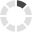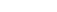Site InformationLoading... Please wait...

DEVRY

•ECET 230 Week 6 iLab Traffic Light Controller Operation

•ECET230 Week 4 iLab Introduction to Flip-Flops Objectives:  Simulate an edge-triggered D flip-flop.  Test a 74LS74 D flip-flop and compare against predictions.  Describe and simulate edge-triggered D and...

•ECET 230 Week 3 iLab Designing Adders and Subtractors Objectives: 1.         To understand the use of BIT and INTEGER data types in VHDL. 2...

•ECET 330 Week 7 iLab Embedded C Programming: Part II Objectives:   1. To become familiar with enabling internal pull-up resistors and reading an input port 2. To learn how to wait for a pushbutton press 3. To learn how...

•ECET230 Week 2 iLab Decoders and Multiplexers Objectives: Discover the operation of 7-segment displays, BCD-to-7-semgment decoders, multiplexers and demultiplexers.  Demonstrate the simulation of a discrete DEMUX...

•ECET 230 Week 1 iLab Introduction to Quartus II, VHDL, and the FPGA Board Objectives: 1.Learn How to write basic logic circuits using VHDL. 2.Using Quartus II compile and simulate the text file and then analyze the...

•ECET 330 Week 7 Homework 1. Is it possible to interface an IC with a different technology such as TTL to HCS12 ports? What are the conditions in terms of electrical parameters that need to be satisfied for this purpose?...

•ECET 230 Homework Assignment 7 1. Is the state machine below a Moore machine or a Mealy machine? Explain your rationale. 3. Using the state diagram in Figure 10.44 on page 663 of the Dueck textbook, briefly explain the...

•ECET 330 Week 6 iLab Embedded C Programming: Part I Objectives:   1. To learn how to write a C program using CodeWarrior IDE 2. To learn how to program I/O instructions in C language 3. To learn how to organize a C...

•ECET 230 Homework Assignment 6 1.The group of bits 10110101 is serially shifted (right-most bit first) into an 8-bit shift register with an initial state of 11100100. After two clock pulses, the register contains: (a)...

•ECET 330 Week 6 Homework 1. Write a program to continuously read the DIP switches connected to PORTA and send it to PORTB. 2. Assume that eight DIP switches are connected to PORTB and eight LEDs are connected to...

•ECET 230 Homework Assignment 5 1.Using Quartus II, or an equivalent VHDL entry program, develop the text file and simulation for the circuit below. Attach the .vhd and simulation files. 2.What is the output frequency of Q1...

•ECET 230 Homework Assignment 4 1. Sketch the Q output for the waveforms shown below applied to an active-LOW S-R latch. Assume that Q starts LOW. 2. Sketch the Q output for the waveforms shown. Assume that Q starts LOW. 3...

•ECET 330 Week 5 iLab Data Manipulation in Assembly Language Objectives:   1. Given an arithmetic equation or data conversion, develop an assembly-language algorithm to implement the correct sequence of operations. 2...

•ECET 230 Homework Assignment 3 1.Determine the decimal value of each of the following unsigned binary numbers: 2.Determine the decimal value of each of the following signed binary number displayed in the...

•ECET 330 Week 5 Homework 1. Show the value of the register and the carry after the execution of the ASLA instruction. Given the original content of Register A and carry flag 0   1 0 0 ...

•ECET 230 Homework Assignment 2 1. When a HIGH is on the output of the decoding circuit below, what is the binary code appearing on the inputs? 2. Write the Boolean equations for each of the following codes if an active-LOW...

•ECET 330 Week 4 iLab Introduction to Tower Module Objectives:   1. To set up the Tower System 2. Become familiar with programming and using the Tower Module 3. Become familiar with I/O port programming   Results:...

•ECET 230 Homework Assignment 1 1. Develop the Boolean equation for the circuit shown below 2. Determine the output Y in Problem 1 for the input values shown below 3. Redraw the circuit in Problem 1 using only 2-input NAND...

•ECET 330 Week 4 Homework 1. Write a program to get eight-bit data from PORTA and send it to PORTB and PORTC. Make sure to define each port as input or output 2. Write a program to toggle all bits of PORTA...